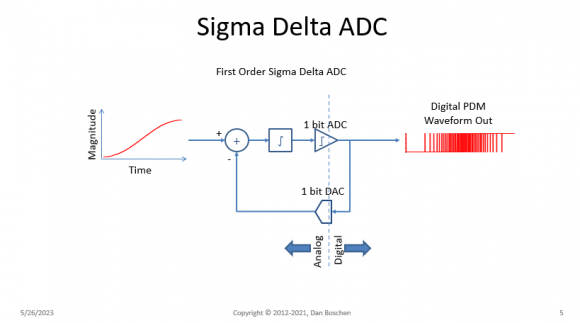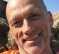## Sigma-delta DAC or delta-sigma DAC?Started by 4 months ago5 replieslatest reply 4 months ago234 views

Which is more correct: sigma-delta DAC or delta-sigma DAC?

If both are valid forms and there are differences, I would like to know that too.

[ - ]To my knowledge both are familiar but Delta-Sigma seems more matched to the functional description of the convertor (make a difference and then average for spectral shaping and down sampling.

Cheers

Laurent

[ - ]The Sigma is the accumulator (for Sigma-Delta DAC) or integrator (for Sigma-Delta ADC as shown below) just prior to decision and feedback. The output of the decision as a pulse-density modulated signal is then followed by the averaging for decimation that you describe, but that isn't the "Sigma" in Delta-Sigma. (To my understanding).[ - ]They are both the same thing and used interchangeably. I must admit that I use both depending on how I wake up in the morning. My impression is Sigma-Delta is more commonly used, but I understand the weak argument that "Delta-Sigma" would be better given it was the first published use to describe such a modulation (Inose 1962) and if you read an implementation from left to right, you first have a Delta as the subtraction followed by the Sigma as an accumulator or integrator. This is an interesting and related read:

[ - ]Common question to ponder...they are both fine. It is a sort of which came first, the chicken or the egg question. I agree with all of the responses. Pavan, Scherier, and Temes famous text on the topic of "such" modulators had this to say on the topic and they say it's delta-sigma. I think I'll leave it at that.

*****************************************

A (friendly) bone of contention in the design community is the choice of nomenclature. Should this be called a delta-sigma modulator, or a sigma-delta modulator? Some designers argue that sigma-delta is apt due to the following. From Figure 2.25(a), we see that the delta operation occurs first, followed by the sigma. In science and engineering, a composite operation is named in the reverse order of the individual operations. For example, to determine the root mean-square value of a waveform, we first square it, determine its mean and then compute the result’s square root. In a similar vein, therefore, this should be called a sigma-delta modulator. The proponents of delta-sigma argue that the modulator historically resulted from cascading an integrator (sigma ) and a (delta) modulator. Going by the reverse-order convention, it makes sense to call this the delta-sigma modulator. Another convention is that the first name used in the literature is the one that should be used, and since Inose and Yasuda chose the name delta-sigma, that is the convention we have adopted. Besides, delta-sigma simply sounds better.

***********************************************

Oh, and that text is called "Understanding Delta-Sigma Converters"

ISBN: 978-1-119-25827-8

[ - ]